## 应用下载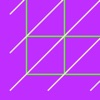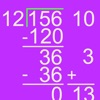This math app can be used to teach a..
This math app can be used to teach and study the partial quotients division method. The app is easy to use and it has an intuitive interactive interface with customizable colors an..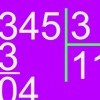The European Long Division app can b..
The European Long Division app can be used to teach and study the long division method that is used in several European countries. The user can solve custom and random division pro..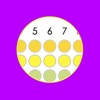Explore and learn the multiplication..
Explore and learn the multiplication table in a visual way. Wired.com: "I highly recommend it for any kid who is learning their multiplication tables". Four different ways to study..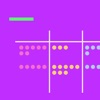Understanding the concept of places ..
Understanding the concept of places and values in the base then system is essential for long-term understanding. In subtraction place value is essential to the concept of regroupin..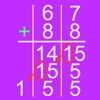This app can be used to teach and st..
This app can be used to teach and study the alternative column-addition method used in the Everyday Mathematics curriculum developed by the University of Chicago School Mathematics..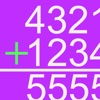This math app can be used to teach a..
This math app can be used to teach and study the column addition method. The app is easy to use and it has an intuitive interactive interface with customizable colors and other set..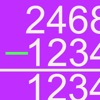This math app can be used to teach a..
This math app can be used to teach and study the column subtraction method. The app is easy to use and it has an intuitive interactive interface with customizable colors and other ..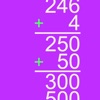The counting-up subtraction app lets..
The counting-up subtraction app lets students solve subtraction problems by counting up from the lesser number step by step. The first step is to get to the nearest multiple of 10...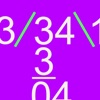The Dutch Long Division app can be u..
The Dutch Long Division app can be used to teach and study the Dutch long division method. The user can solve custom and random division problems with small and large numbers. Endo..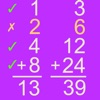This app can be used to teach and st..
This app can be used to teach and study the ancient Egyptian multiplication method. The Egyptian Multiplication Method Ancient Egyptians were able to multiply any two numbers by us..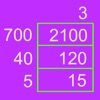This math app can be used to teach a..
This math app can be used to teach and study the grid multiplication method. The app is easy to use and it has an intuitive interactive interface with customizable colors and other..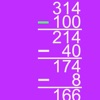This math app can be used to teach a..
This math app can be used to teach and study the left-to-right subtraction method. The app is easy to use and it has an intuitive interactive interface with customizable colors and..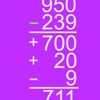This math app can be used to teach a..
This math app can be used to teach and study the partial differences subtraction method. The app is easy to use and it has an intuitive interactive interface with customizable colo..This math app can be used to teach a..
This math app can be used to teach and study the partial products multiplication method. The app is easy to use and it has an intuitive interactive interface with customizable colo..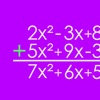With the Polynomial Addition and Sub..
With the Polynomial Addition and Subtraction app you can practice adding and subtracting polynomials with a step-by-step method. And you can get additional help at each step. The a..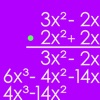With the Polynomial Multiplication a..
With the Polynomial Multiplication app you can practice multiplying polynomials with a step-by-step method. And you can get additional help at each step. The app saves your most re..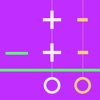In the Same-Change Subtraction app t..
In the Same-Change Subtraction app the user can keep adding or subtracting the same amount from both numbers until the subtraction problem can be solved easily. The same-change rul..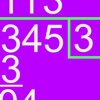The Swedish Long Division app can be..
The Swedish Long Division app can be used to teach and study the Swedish long division method. The user can solve custom and random division problems with small and large numbers. ..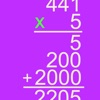This math app can be used to teach a..
This math app can be used to teach and study the partial products multiplication method. The app is easy to use and it has an intuitive interactive interface with customizable colo..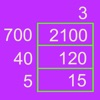This math app can be used to teach a..
This math app can be used to teach and study the grid multiplication method. The app is easy to use and it has an intuitive interactive interface with customizable colors and other..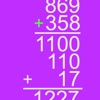This app can be used to teach and st..
This app can be used to teach and study the partial sums addition method. The app is easy to use and it has an intuitive interactive interface with customizable colors and other se..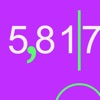This math app can be used to teach a..
This math app can be used to teach and study the rounding of whole numbers. You can set your own problems or solve random problems. The numbers to be rounded can have up to 8 digit..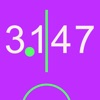This math app can be used to teach a..
This math app can be used to teach and study the rounding of decimals. You can set your own problems or solve random problems. The decimal numbers can be rounded to nearest whole n..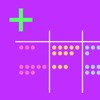Understanding the concept of places ..
Understanding the concept of places and values in the base then system is essential for long-term understanding. In addition place value is essential to the concept of regrouping. ..